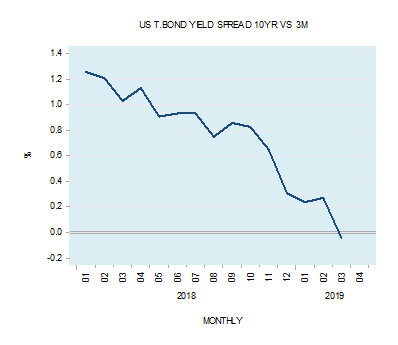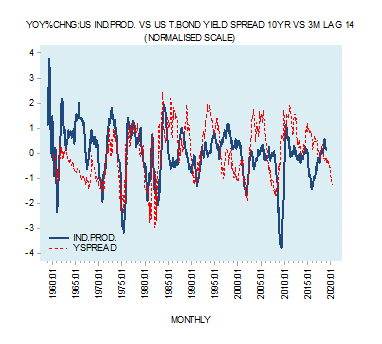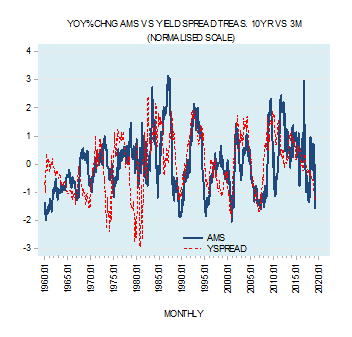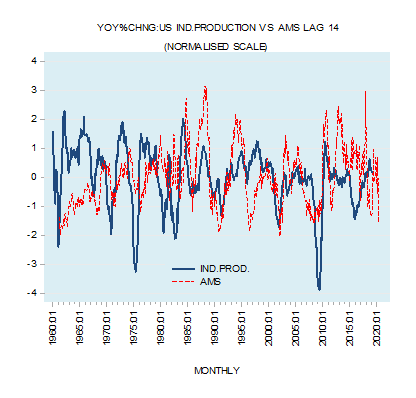What the shape of the yield spread implies?

A fall in the difference between the yield on the 10-year US T-Bond and the US 3-month Treasury bill into negative territory has prompted many commentators to suggest that this points to a likely economic recession in the months ahead. The difference stood at minus 0.043% in March against 0.271% in February and 1.029% in March last year.Historically, most recessions in the US were preceded by significant declines in the differential between the 10-year T-bond and the 3-month Treasury security. Typically, this narrowing in the spread occurs many months before the onset of the recession.

Conversely, the widening in the spread occurs many months before the beginning of the economic recovery. What is the reason for that?The conventional view

The most popular explanation for what determines the shape of the yield curve is provided by expectation theory (ET). According to ET, the key to the shape of the yield curve is that long-term interest rates are the average of expected future short-term rates.

If today’s one year rate is 4% and next year’s one year rate is expected to be 5%, then the two year rate today should be 4.5% (4+5)/2=4.5%. Here the long-term rate, i.e. the two year rate today, is higher than the short term (i.e. one year) rate.

It follows then that expectations for rises in short-term rates will make the yield curve upward sloping, as long-term rates will be higher than short-term rates. Conversely, expectations for a decline in short-term rates will result in a downward sloping yield curve, as long-term rates will be lower than short-term rates.

If today’s one year rate is 4% and next year’s one year rate is expected to be 3%, then the two year rate today should be 3.5% (4+3)/2=3.5%. The long-term rate (i.e. the two year rate today) is lower than the short term.

According to ET followers, whenever investors expect economic expansion they will also start expecting rising short-term interest rates. Consequently, following the ET way of thinking the long-term interest rate is going to be higher than the short-term interest rate (remember according to ET the long-term interest rate today is an average of today’s and expected short-term interest rates). Hence, an upward sloping yield curve emerges.

Conversely, an economic slump is associated with expectations for a falling short – term interest rates. As a result, according to ET, the long-term interest rate today will be lower than the short-term interest rate – a downward slopping yield curve emerges

Note that in this framework of thinking the formation of expectations regarding short term interest rates in accordance with the expected economic environment determines long term interest rates and in turn the shape of the yield curve.

Shape of the yield curve in an unhampered market

In his writings, Murray Rothbard argued that in a free, unhampered market economy an upward sloping yield curve cannot be sustained because it would set in place an arbitrage between short and long-term securities. This would lift short-term interest rates and lower long-term interest rates, resulting in the tendency towards a uniform interest rate throughout the term structure. Arbitrage will also prevent the sustainability of a downward sloping yield curve by shifting funds from long maturities to short maturities and thereby flattening the curve.

Similarly, Ludwig von Mises concluded that in a free, unhampered market economy the natural tendency of the shape of the yield curve is neither towards an upward slope nor towards a downward slope but rather towards flattening.

On this Mises wrote,

The activities of the entrepreneurs tend toward the establishment of a uniform rate of originary interest in the whole market economy. If there turns up in one sector of the market a margin between the prices of present goods and those of future goods which deviates from the margin prevailing on other sectors, a trend toward equalization is brought about by the striving of businessmen to enter those sectors in which this margin is higher and to avoid those in which it is lower. The final rate of originary interest is the same in all parts of the market of the evenly rotating economy.

Following Mises and Rothbard (MR) we suggest that in a free, unhampered market economy a prolonged upward or downward sloping yield curve cannot be sustained. What then is the mechanism that generates a sustained upward or downward sloping yield curve?

MR have concluded that this must be the result of the central bank tampering with financial markets by means of monetary policies.

How the Fed’s tampering generates an upward or downward sloping yield curve

While the Fed can exercise control over short-term interest rates via the federal funds rate, it has lesser control over longer-term rates. In this sense, long-term rates can be seen as reflecting, to a greater degree, the underlying time preferences of individuals.

The Fed’s monetary policies disrupt the natural tendency towards uniformity of interest rates along the term structure.  This disruption leads to the deviation of short-term rates from the natural rate i.e. from individuals’ time preferences as partially mirrored by the relatively less-manipulated long-term rate.

When the Fed lowers the policy interest rate target this almost instantly lowers short-term interest rates, whilst to a lesser degree affecting longer-term rates. As a result, an upward sloping yield curve develops. (The interest rate differential between the long-term rate and the short-term rate widens).

Conversely, when the Fed reverses its stance and lifts the policy interest rate target this lifts short-term interest rates. As a result, a downward sloping yield curve emerges. (The differential between the long-term rate and the short-term rate narrows).

As a rule Fed policy makers decide about their interest rate stance in accordance with the observed and expected state of the economy and price inflation. Whenever the economy starts to show signs of weakening and the rate of increase of various price indexes also begins to soften, investors in the market begin to form expectations that in the months ahead the Fed is going to lower its policy interest rate.

As a result, short term interest rates also begin to move lower. The spread between long term rates and short term rates widens – the process of the development of an upward sloping yield curve is set in motion. However this process cannot be sustained unless the Fed actually lowers the policy interest rate. Once the Fed has lowered the policy rate the widening in the yield spread gets consolidated.

Conversely, whenever economic activity shows signs of strengthening, coupled with a rise in price inflation, investors’ in the market start to form expectations that in the months ahead the Fed is going to lift its policy interest rate.

As a result short term interest rates begin to move higher. The spread between long term rates and short term rates narrows – the process of the development of a downward sloping yield curve is set in motion. Also here this process cannot be sustained without the Fed’s actually raising the policy interest rate. Once the Fed raises the policy interest rate, the narrowing in the yield spread consolidates.

Note that using MR ideas we can suggest that an upward sloping yield curve is the result of investors’ expectations of an easy interest rate policy. These expectations are then followed by the central bank actually easing the policy interest rate. This is contrary to the ET framework where the upward sloping curve emerges on account of investors expecting an increase in short term interest rates.

With respect to a downward sloping yield curve, we suggest that it emerges as a result of investors’ expectations of a tighter interest rate stance by the central bank. These expectations are then followed by the central bank actually raising the policy interest rate. Again, this is contrary to the ET framework where the downward sloping yield curve emerges on account of investors’ expectations of a decline in short term interest rates.

Why do changes in the shape of the yield curve precede economic activity?

Whenever the central bank reverses its monetary stance and alters the shape of the yield curve it sets in motion either an economic boom or an economic bust.  However these booms and slumps arise with time lags – they are not immediate. The reason for this is the fact that the effect of a change in monetary policy works with a time lag as the effect shifts from one market to another market, from one individual to another individual.

It is this lagged effect of a change in monetary policy that makes the change in the shape of the yield curve a good predictive tool.  For instance, when, during an economic expansion, the central bank raises its interest rates and flattens the yield curve, the effect is minimal as economic activity is still dominated by the previous easy monetary stance. It is only later on, once the tighter stance begins to dominate the scene that economic activity begins to weaken.

Yield spread and AMS annual growth rate

Note that fluctuations in the yield spread are closely associated with fluctuations in the annual growth rate of AMS (see chart).Thus, an upward slopping yield curve as a result of the lowering of short-term interest rates by the Fed is associated with a strengthening in the generation of money out of thin air and in the strengthening in the momentum of AMS.

A downward slopping yield curve due to a tighter interest rate stance of the Fed is associated with a weakening in the pace of creation of money out of thin air and hence with a decline in the annual growth rate of AMS.

This of course means that fluctuations in the momentum of AMS that corresponds to the relevant yield spreads provide signals regarding future fluctuations in economic activity.

Based on the current downward slopping yield curve and the downward momentum of AMS we can suggest that the annual growth rate of economic activity in terms of industrial production is likely to come under pressure in the months ahead (see chart).Summary and conclusion

According to the Expectations Theory (ET) framework, the downward sloping yield curve emerges in response to the expectations of a decline in short-term interest rates. In the Mises – Rothbard (MR) framework, the downward sloping yield curve emerges because of a tighter interest rate stance by the Fed.

The upward sloping yield curve emerges in the ET framework in response to expectations of an increase in short-term interest rates. In the MR framework, the upward sloping yield curve is due to a lowering of the policy interest rate – the exact opposite of the ET.

Furthermore, we can also suggest that the shape of the yield curve corresponds to the shape of the growth momentum of AMS.

Consequently, based on a prolonged decline in the growth momentum of AMS we can suggest that the annual growth rate of US industrial production is likely to come under continued downward pressure in the months ahead.

 Murray Rothbard Man, Economy, and State Nash Publishing p 384

 Ludwig von Mises Human Action third edition p536

Latest Posts By